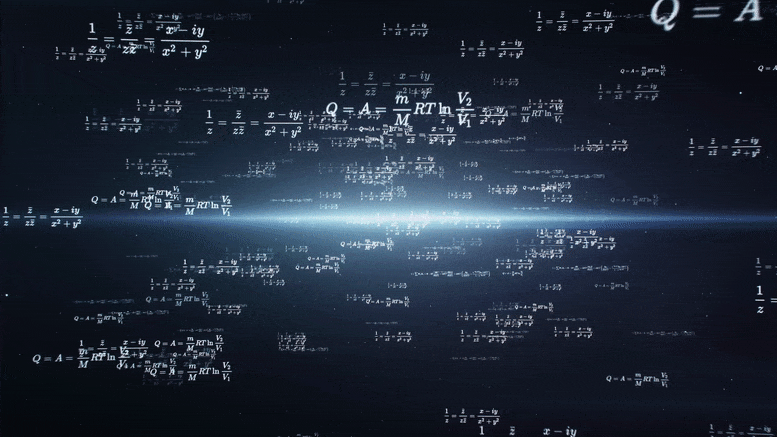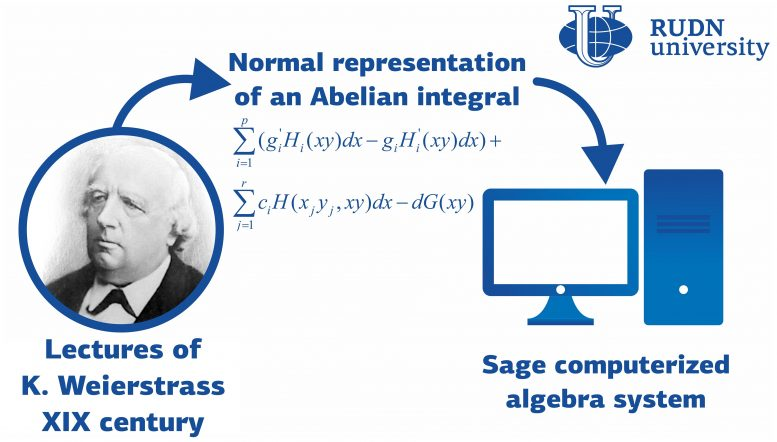# Mathematicians Apply 19th Century Ideas to Modern Computerized Algebra SystemsA team of mathematicians from RUDN University added new symbolic integration functionality to the Sage computerized algebra system. The team implemented ideas and methods suggested by the German mathematician Karl Weierstrass in the 1870s. The results were published in the Journal of Symbolic Computation.

The first computer program capable of calculating integrals of elementary functions was developed in the late 1950s. By creating it, the developers confirmed that a computer could not only perform simple calculations but was also able to deal with tasks that required a certain degree of ‘thinking.’ Symbolic integration, i.e. integration that involves letters and abstract symbols instead of numbers, is an example of such a task.

At the same time, scientists realized that neither humans nor computers were able to determine whether a given integral can be taken in elementary functions (provided such a human or computer used the methods studied in a university course of analysis and took a finite number of steps). Therefore, in the 1960s mathematicians working on symbolic integrators started to refer to methods that had been suggested by Liouville in the 1830s. From that time on, computer scientists have been tapping into the classic scientific heritage.A team of mathematicians from RUDN University added new symbolic integration functionality to the Sage computerized algebra system. The team implemented ideas and methods suggested by the German mathematician Karl Weierstrass in the 1870s. Credit: RUDN University

The calculation of primitives of algebraic functions is one of the bottlenecks in the process of integrator development. Before World War I, the integration of algebraic functions or Abelian integrals had been considered one of the most important issues in mathematics, but later on, it was forgotten.

“Current computer algebra systems are able to fulfill even the most exotic requests of mathematical analysis students, but at the same time, many of these systems fail to recognize integrals in elementary functions. Only several packages allow for the integration of algebraic functions or with Abelian integrals, but their development stopped 15 years ago, and their functionality leaves much to be desired,” says Mikhail Malykh, a Doctor of Science in Physics and Mathematics, and an assistant professor at the Department of Applied Informatics and Probability Theory, RUDN University.

One of the theories developed by the German mathematician Karl Weierstrass in the 1870s reduces the calculation of an integral of an algebraic function to finding a given set of known integrals of all three types. The initial integral is represented as a sum of standard integrals (this construction is knowns as the normal representation of an Abelian integral). The team from RUDN University confirmed that this representation is indicative of whether a given integral can be calculated in elementary functions. To confirm their theory, the mathematicians tested them on simple elliptical integrals using a software package that had been created by the team in 2017. The package helps calculate coefficients of the normal form of an integral. In the future, the team plans to conduct similar studies for a wider range of integrals.

“This work is just one step on our way to an ambitious goal: we want to express Weierstrass’s theory of Abelian integrals and functions using the language of computer algebra and to implement it in the Sage system, giving researchers from all over the world free access to it,” added Mikhail Malykh from RUDN University.

Reference: “On symbolic integration of algebraic functions” by M.D. Malykh, L.A. Sevastianova and Y. Yu, 11 September 2020, Journal of Symbolic Computation.
DOI: 10.1016/j.jsc.2020.09.002

#### 1 Commenton "Mathematicians Apply 19th Century Ideas to Modern Computerized Algebra Systems"

1.bruce | December 1, 2020 at 11:16 am | Reply

this is good for my condone merch bdeoliln